# Training accesories

31 products found
Filtres

Gender

Size

Price

€10.00 - €40.00

Couleurs

Sport

Use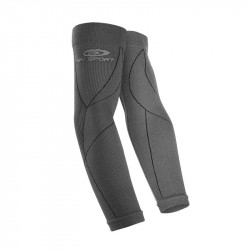• S/M
• L/XL• S/M
• L/XL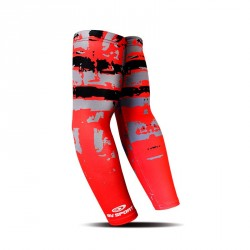• L/XL• L/XL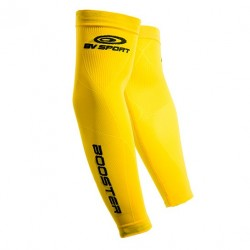• L/XL• L/XL• L/XL• S/M
• L/XL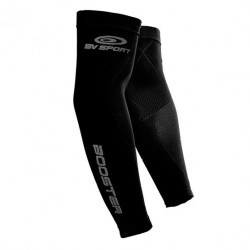• S/M
• L/XL• L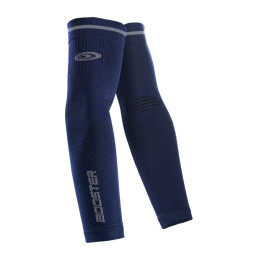• S
• M
• L• S
• M
• L• S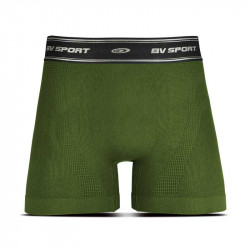• S• One Size• One Size• One Size• One Size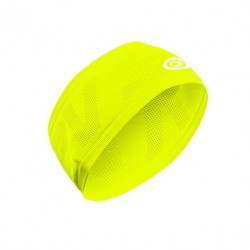• One Size• One Size• One Size• One Size• One Size• One Size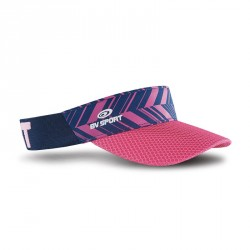• One Size• One Size• S
• M
• L
• XL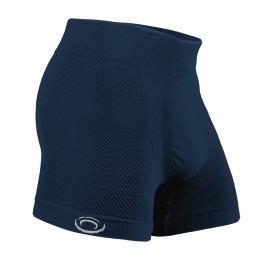• S
• M
• L• One Size